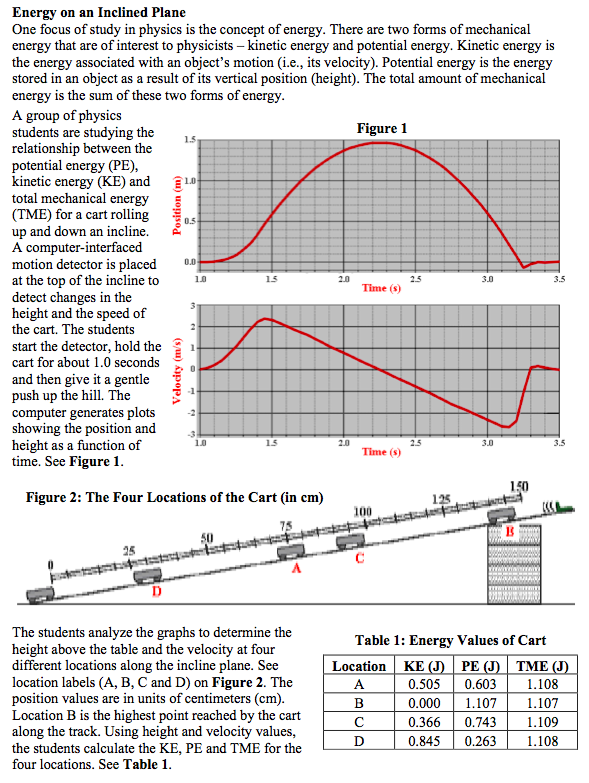###Energy on an Inclined Plane

Resource:
Science Reasoning Center: Energy on an Inclined Plane

Description:
This passage describes an experiment in which students use a motion detector to study the changes in the kinetic and potential energy of a cart that rolls up and down an inclined plane. Information is presented in the form of a position-time graph, a velocity-time graph, a figure and a table. Questions target a student's ability to read values off of graphs, to use information from one graph to answer questions about another graph or a table, to draw conclusions that are consistent with the presented data, and to use presented data to predict the result of performing an additional trial.

Performance Expectation:
HS-PS3-2  Develop and use models to illustrate that energy at the macroscopic scale can be accounted for as a combination of energy associated with the motions of particles (objects) and energy associated with the relative positions of particles (objects).

This activity aligns with the three dimensions of the Next Generation Science Standards in the manner described below:

Disciplinary Core Ideas
 Definitions of Energy (HS-PS3.A.1): Energy is a quantitative property of a system that depends on the motion and interactions of matter and radiation within that system. That there is a single quantity called energy is due to the fact that a system's total energy is conserved, even as, within the system, energy is continually transferred from one object to another and between its various forms. In this passage, a cart’s position and speed on a ramp and are used to calculate the potential energy, kinetic energy, and total energy of the system (cart). The student will recognize that for this closed system the total energy of the system is conserved.  And while energy is transferred from kinetic energy to potential energy and then back to kinetic energy, the total mechanical energy (TME) remains constant.

Crosscutting Concepts
 System and System Model: Models (e.g., physical, mathematical, computer models) can be used to simulate systems and interactions—including energy, matter, and information flows—within and between systems at different scales. Two computer-generated graphs—cart position and cart velocity as functions of time—help the student understand relationships between the types of energy in a closed system and the total energy.  A data table at the end of the data presentation illustrates the potential energy, kinetic energy, and total energy at four locations during the cart’s motion.  Despite the fact that the potential energy and kinetic energy vary from one location to another, the reader can see that the total energy of the cart system remains unchanged. Energy and Matter: The total amount of energy and matter in closed systems is conserved. As one of the great laws of science, conservation of energy can be clearly understood in this simple system.  The student will see that although energy can be transferred from one type to another, the total amount of energy in the cart system remains unchanged.  This data presentation serves as a simple yet powerful empirical way of helping students grasp the meaning and significance of this great conservation law.

Practices
 Analyzing and Interpreting Data:  Analyze data using computational models in order to make valid and reliable scientific claims. Using data collected by computer-based motion sensors, students consider both the ‘raw’ data of a cart’s position and velocity as a function of time as well as the calculated quantities of the cart’s kinetic energy, potential energy and total energy.  As students consider the position and velocity graphs, they understand how these quantities are integral in determining the types of energy present.   Several questions at the end of the passage also lead students to ‘discover’ the important concept that although the cart slows down and speeds up as it moves up and down the ramp the total energy of the system stays constant. Engaging in Argument from Evidence: Apply scientific principles and evidence to provide an explanation of phenomena and solve design problems, taking into account possible unanticipated effects. After understanding how conservation of energy manifests itself in this experiment, students are asked to apply this scientific principle to new situations related to the scenario at hand.  The last several questions in this passage are examples of these extensions in which students are asked to engage in argument from the evidence uncovered in the first set of questions. Using Mathematical and Computational Thinking: Use mathematical and/or computational representations of phenomena or design solutions to support explanations. Questions 3, 4 and 9 at the end of the passage ask students to use mathematical thinking to understand how position and velocity are related to the quantities of potential and kinetic energy.  It is through these computations that students begin to understand why energy is such an important quantity in science in that it is the quantity that is conserved in a closed system.

Associated Reading from The Physics Classroom

Other Supporting Pages at The Physics Classroom:

View Infographic.
(Coming Soon)

### Search the NGSS Corner

Maybe you're looking for something really specific that pertains to a desired topic and emphasizes one or more of the listed NGSS dimensions. Why not try a search of this section of our website? Simply select from one or more of the pull-down menus and click Search  This page will reload and a collection  of possibilities will be displayed in this section of the page and sorted by relevancy.